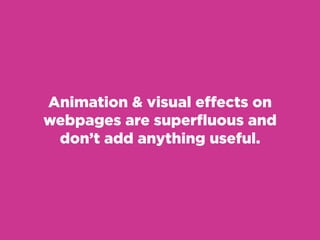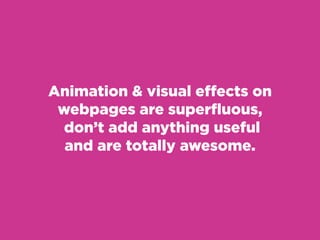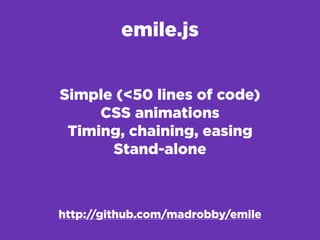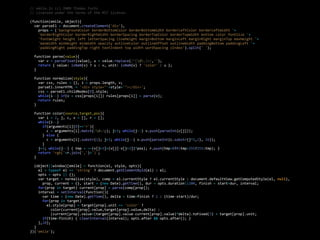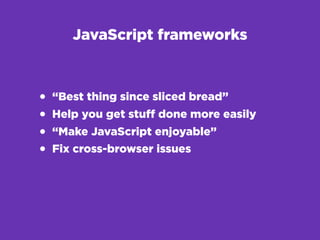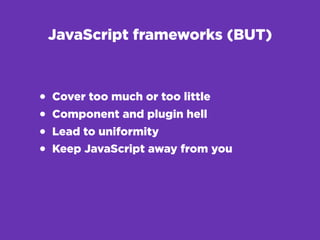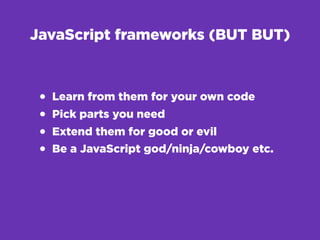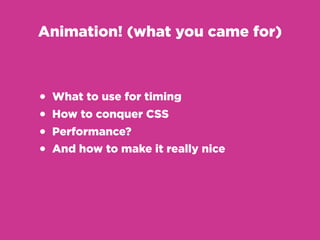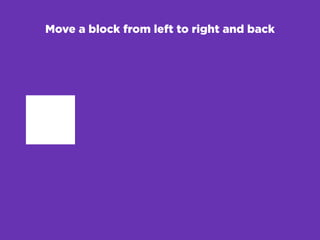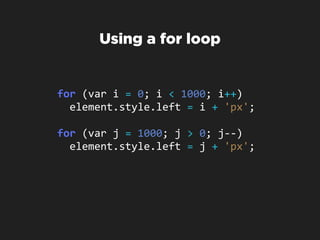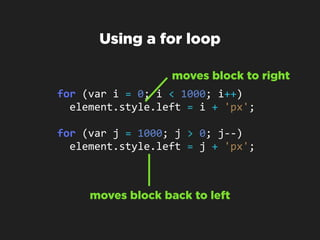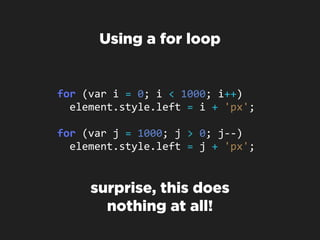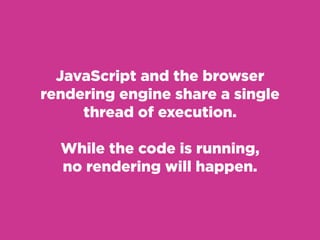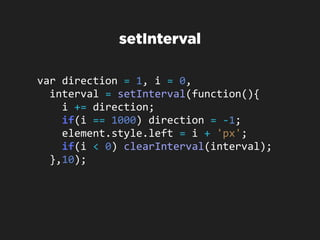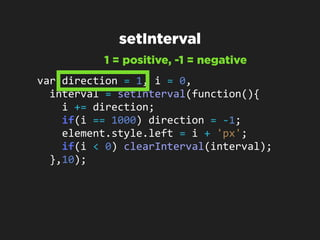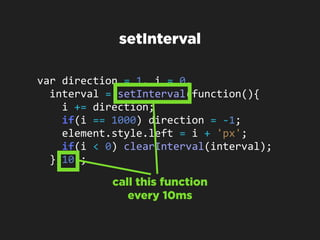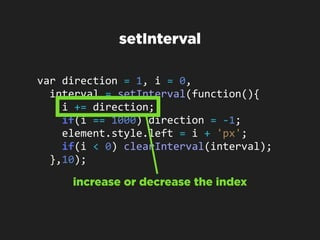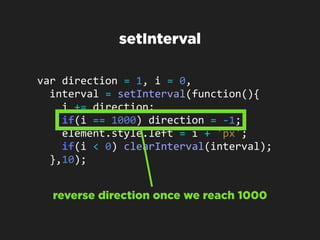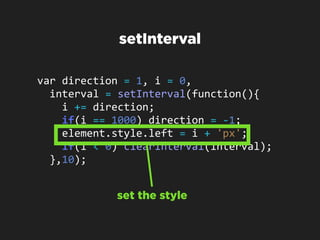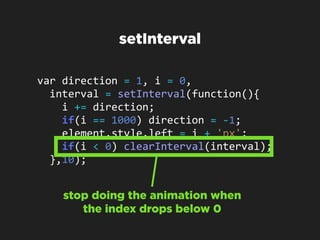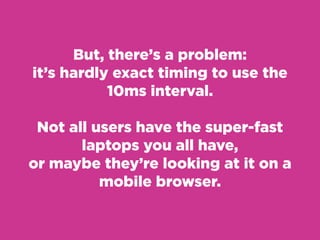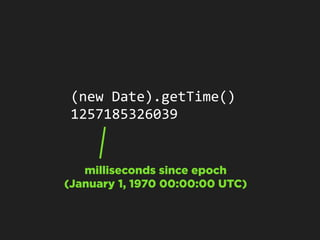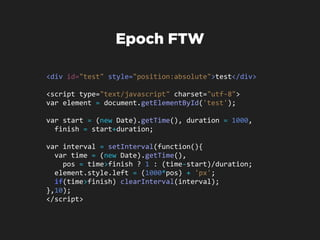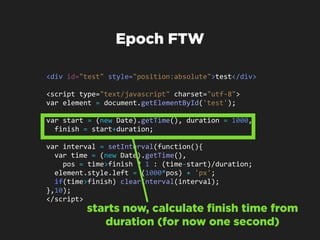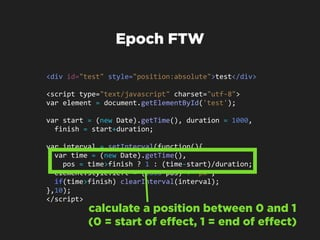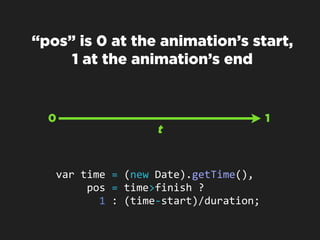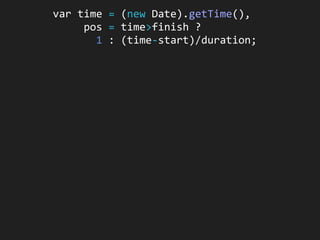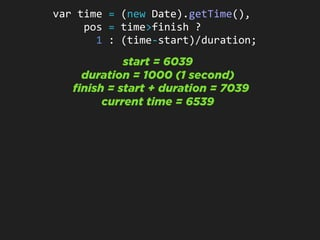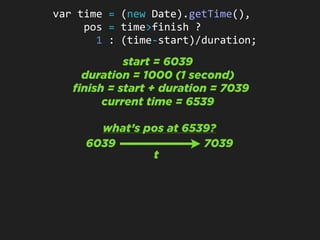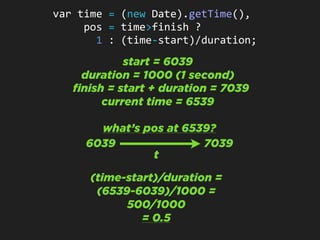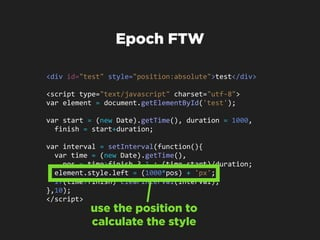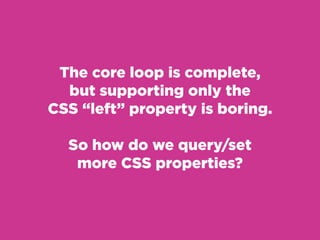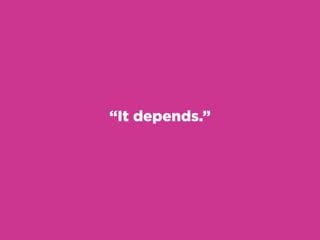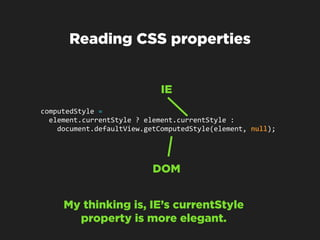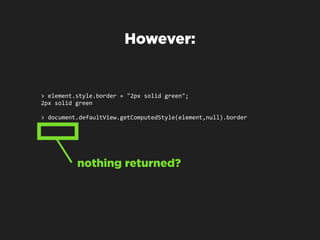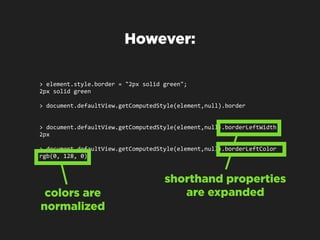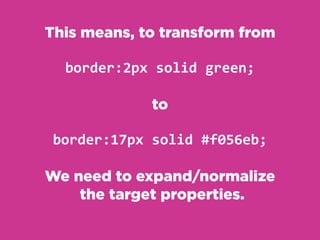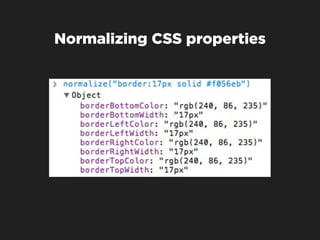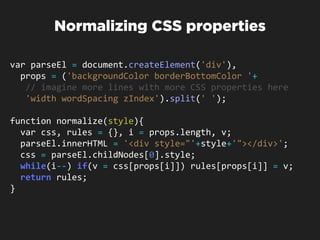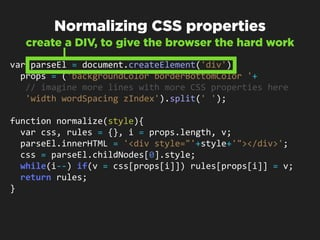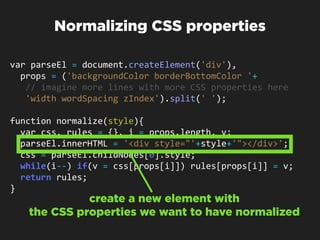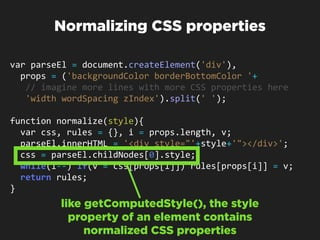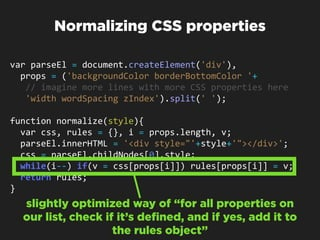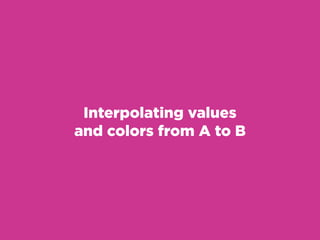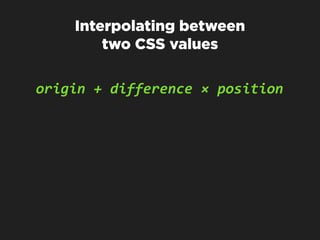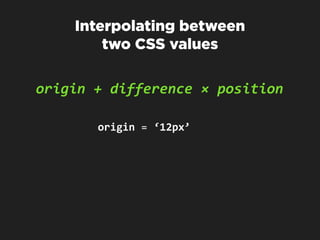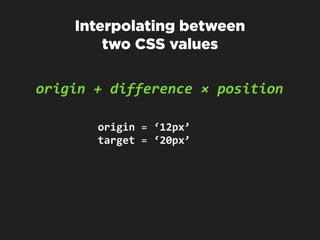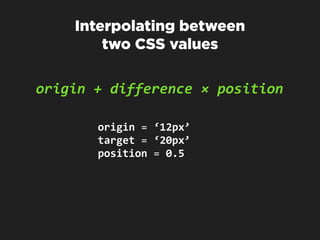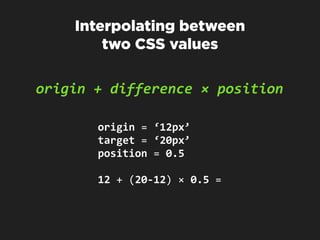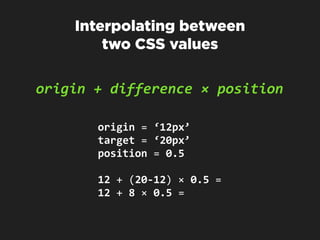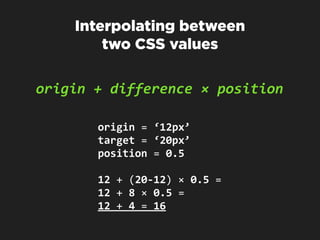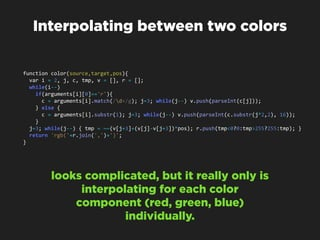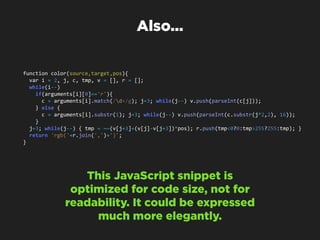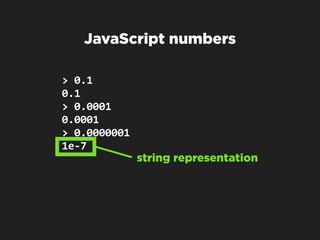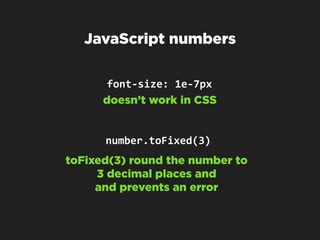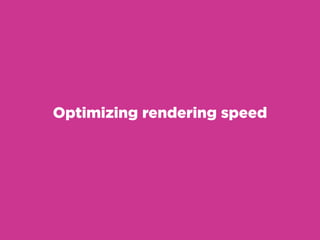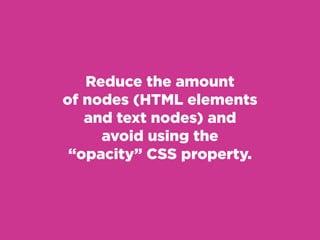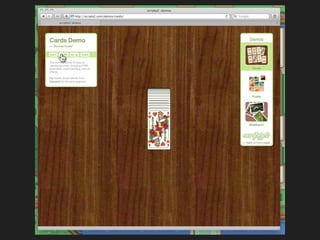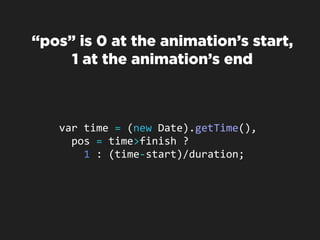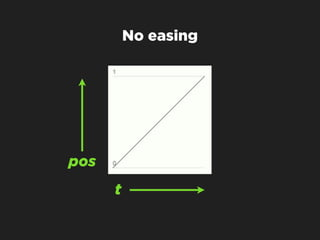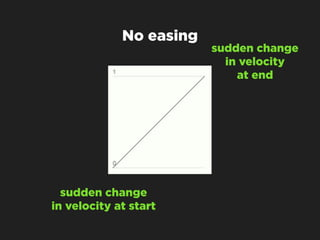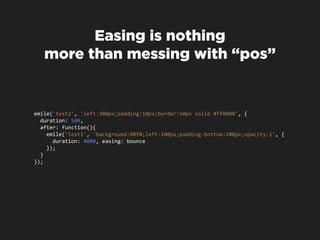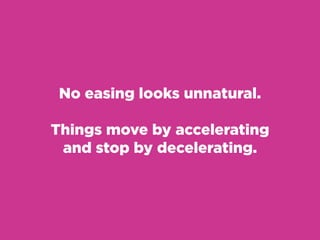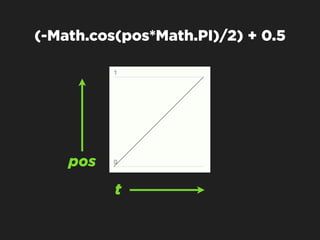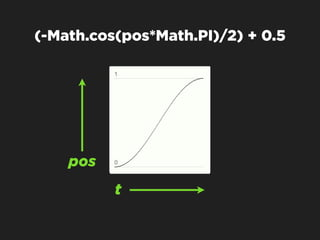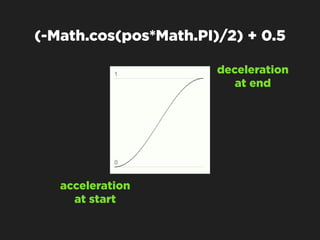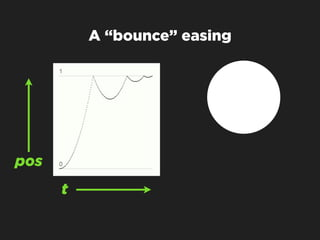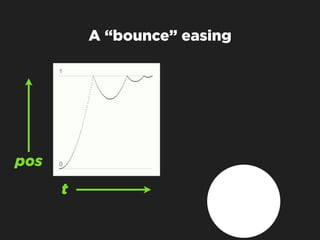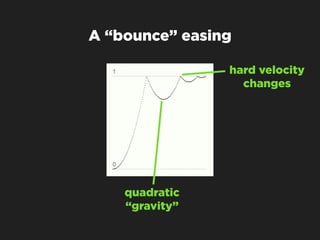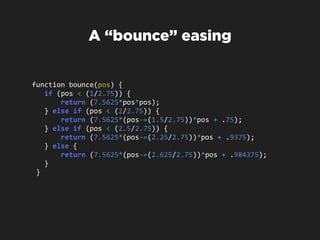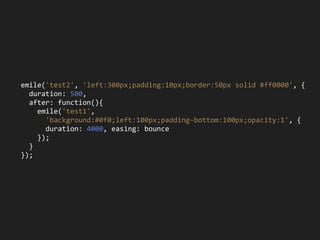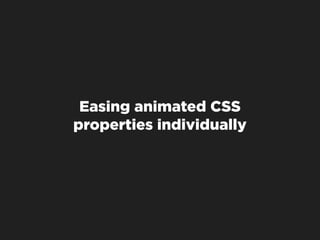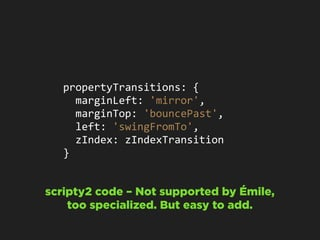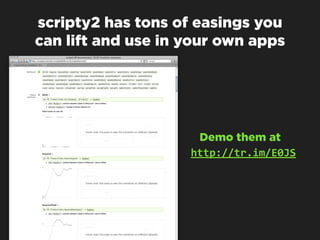1 of 87

### Émile - a JavaScript animation framework in 50 lines of code

1. Thomas Fuchs Roll your own JavaScript Effects Framework (and introducing Émile)
2. Émile Cohl “The father of the animated cartoon” http://en.wikipedia.org/wiki/File:Young_Emile_Cohl.jpg
4. Animation & visual effects on webpages are superfluous and don’t add anything useful.
5. Animation & visual effects on webpages are superfluous, don’t add anything useful and are totally awesome.
6. Animation & visual effects on webpages are superfluous, don’t add anything useful and are totally awesome. what’s important to the user?
7. emile.js Simple (<50 lines of code) CSS animations Timing, chaining, easing Stand-alone http://github.com/madrobby/emile
8. //  emile.js  (c)  2009  Thomas  Fuchs //  Licensed  under  the  terms  of  the  MIT  license. (function(emile,  object){    var  parseEl  =  document.createElement('div'),        props  =  ('backgroundColor  borderBottomColor  borderBottomWidth  borderLeftColor  borderLeftWidth  '+        'borderRightColor  borderRightWidth  borderSpacing  borderTopColor  borderTopWidth  bottom  color  fontSize  '+        'fontWeight  height  left  letterSpacing  lineHeight  marginBottom  marginLeft  marginRight  marginTop  maxHeight  '+        'maxWidth  minHeight  minWidth  opacity  outlineColor  outlineOffset  outlineWidth  paddingBottom  paddingLeft  '+        'paddingRight  paddingTop  right  textIndent  top  width  wordSpacing  zIndex').split('  ');    function  parse(value){        var  v  =  parseFloat(value),  u  =  value.replace(/^[d.]+/,'');        return  {  value:  isNaN(v)  ?  u  :  v,  unit:  isNaN(v)  ?  'color'  :  u  };    }    function  normalize(style){        var  css,  rules  =  {},  i  =  props.length,  v;        parseEl.innerHTML  =  '<div  style="'+style+'"></div>';        css  =  parseEl.childNodes.style;        while(i-­‐-­‐)  if(v  =  css[props[i]])  rules[props[i]]  =  parse(v);        return  rules;    }        function  color(source,target,pos){        var  i  =  2,  j,  c,  v  =  [],  r  =  [];        while(i-­‐-­‐)              if(arguments[i]=='r'){                c  =  arguments[i].match(/d+/g);  j=3;  while(j-­‐-­‐)  v.push(parseInt(c[j]));            }  else  {                c  =  arguments[i].substr(1);  j=3;  while(j-­‐-­‐)  v.push(parseInt(c.substr(j*2,2),  16));            }        j=3;  while(j-­‐-­‐)  {  tmp  =  ~~(v[j+3]+(v[j]-­‐v[j+3])*pos);  r.push(tmp<0?0:tmp>255?255:tmp);  }        return  'rgb('+r.join(',')+')';    }        (object||window)[emile]  =  function(el,  style,  opts){        el  =  typeof  el  ==  'string'  ?  document.getElementById(el)  :  el;        opts  =  opts  ||  {};        var  target  =  normalize(style),  comp  =  el.currentStyle  ?  el.currentStyle  :  document.defaultView.getComputedStyle(el,  null),            prop,  current  =  {},  start  =  (new  Date).getTime(),  dur  =  opts.duration||200,  finish  =  start+dur,  interval;        for(prop  in  target)  current[prop]  =  parse(comp[prop]);        interval  =  setInterval(function(){            var  time  =  (new  Date).getTime(),  delta  =  time>finish  ?  1  :  (time-­‐start)/dur;            for(prop  in  target)                el.style[prop]  =  target[prop].unit  ==  'color'  ?                    color(current[prop].value,target[prop].value,delta)  :                      (current[prop].value+(target[prop].value-­‐current[prop].value)*delta).toFixed(3)  +  target[prop].unit;            if(time>finish)  {  clearInterval(interval);  opts.after  &&  opts.after();  }        },10);    } })('emile');
9. Wait, hold it! Why write something new from scratch?
10. JavaScript frameworks • “Best thing since sliced bread” • Help you get stuff done more easily • “Make JavaScript enjoyable” • Fix cross-browser issues
11. JavaScript frameworks (BUT) • Cover too much or too little • Component and plugin hell • Lead to uniformity • Keep JavaScript away from you
12. JavaScript frameworks (BUT BUT) • Learn from them for your own code • Pick parts you need • Extend them for good or evil • Be a JavaScript god/ninja/cowboy etc.
13. Animation! (what you came for) • What to use for timing • How to conquer CSS • Performance? • And how to make it really nice
14. Move a block from left to right and back
15. Move a block from left to right and back
16. Using a for loop for  (var  i  =  0;  i  <  1000;  i++)    element.style.left  =  i  +  'px'; for  (var  j  =  1000;  j  >  0;  j-­‐-­‐)    element.style.left  =  j  +  'px';
17. Using a for loop moves block to right for  (var  i  =  0;  i  <  1000;  i++)    element.style.left  =  i  +  'px'; for  (var  j  =  1000;  j  >  0;  j-­‐-­‐)    element.style.left  =  j  +  'px'; moves block back to left
18. Using a for loop for  (var  i  =  0;  i  <  1000;  i++)    element.style.left  =  i  +  'px'; for  (var  j  =  1000;  j  >  0;  j-­‐-­‐)    element.style.left  =  j  +  'px'; surprise, this does nothing at all!
19. JavaScript and the browser rendering engine share a single thread of execution. While the code is running, no rendering will happen.
20. setInterval var  direction  =  1,  i  =  0,      interval  =  setInterval(function(){        i  +=  direction;        if(i  ==  1000)  direction  =  -­‐1;        element.style.left  =  i  +  'px';        if(i  <  0)  clearInterval(interval);      },10);
21. setInterval 1 = positive, -1 = negative var  direction  =  1,  i  =  0,      interval  =  setInterval(function(){        i  +=  direction;        if(i  ==  1000)  direction  =  -­‐1;        element.style.left  =  i  +  'px';        if(i  <  0)  clearInterval(interval);      },10);
22. setInterval var  direction  =  1,  i  =  0,      interval  =  setInterval(function(){        i  +=  direction;        if(i  ==  1000)  direction  =  -­‐1;        element.style.left  =  i  +  'px';        if(i  <  0)  clearInterval(interval);      },10); call this function every 10ms
23. setInterval var  direction  =  1,  i  =  0,      interval  =  setInterval(function(){        i  +=  direction;        if(i  ==  1000)  direction  =  -­‐1;        element.style.left  =  i  +  'px';        if(i  <  0)  clearInterval(interval);      },10); increase or decrease the index
24. setInterval var  direction  =  1,  i  =  0,      interval  =  setInterval(function(){        i  +=  direction;        if(i  ==  1000)  direction  =  -­‐1;        element.style.left  =  i  +  'px';        if(i  <  0)  clearInterval(interval);      },10); reverse direction once we reach 1000
25. setInterval var  direction  =  1,  i  =  0,      interval  =  setInterval(function(){        i  +=  direction;        if(i  ==  1000)  direction  =  -­‐1;        element.style.left  =  i  +  'px';        if(i  <  0)  clearInterval(interval);      },10); set the style
26. setInterval var  direction  =  1,  i  =  0,      interval  =  setInterval(function(){        i  +=  direction;        if(i  ==  1000)  direction  =  -­‐1;        element.style.left  =  i  +  'px';        if(i  <  0)  clearInterval(interval);      },10); stop doing the animation when the index drops below 0
27. Much better, as in, there’s actually some animation going on.
28. But, there’s a problem: it’s hardly exact timing to use the 10ms interval. Not all users have the super-fast laptops you all have, or maybe they’re looking at it on a mobile browser.
29. (new  Date).getTime() 1257185326039 milliseconds since epoch (January 1, 1970 00:00:00 UTC)
30. Epoch FTW <div  id="test"  style="position:absolute">test</div> <script  type="text/javascript"  charset="utf-­‐8"> var  element  =  document.getElementById('test'); var  start  =  (new  Date).getTime(),  duration  =  1000,      finish  =  start+duration;     var  interval  =  setInterval(function(){    var  time  =  (new  Date).getTime(),          pos  =  time>finish  ?  1  :  (time-­‐start)/duration;    element.style.left  =  (1000*pos)  +  'px';            if(time>finish)  clearInterval(interval); },10); </script>
31. Epoch FTW <div  id="test"  style="position:absolute">test</div> <script  type="text/javascript"  charset="utf-­‐8"> var  element  =  document.getElementById('test'); var  start  =  (new  Date).getTime(),  duration  =  1000,      finish  =  start+duration;     var  interval  =  setInterval(function(){    var  time  =  (new  Date).getTime(),          pos  =  time>finish  ?  1  :  (time-­‐start)/duration;    element.style.left  =  (1000*pos)  +  'px';            if(time>finish)  clearInterval(interval); },10); </script> starts now, calculate finish time from duration (for now one second)
32. Epoch FTW <div  id="test"  style="position:absolute">test</div> <script  type="text/javascript"  charset="utf-­‐8"> var  element  =  document.getElementById('test'); var  start  =  (new  Date).getTime(),  duration  =  1000,      finish  =  start+duration;     var  interval  =  setInterval(function(){    var  time  =  (new  Date).getTime(),          pos  =  time>finish  ?  1  :  (time-­‐start)/duration;    element.style.left  =  (1000*pos)  +  'px';            if(time>finish)  clearInterval(interval); },10); </script> calculate a position between 0 and 1 (0 = start of effect, 1 = end of effect)
33. “pos” is 0 at the animation’s start, 1 at the animation’s end 0 1 t var  time  =  (new  Date).getTime(),            pos  =  time>finish  ?                1  :  (time-­‐start)/duration;
34. var  time  =  (new  Date).getTime(),            pos  =  time>finish  ?                1  :  (time-­‐start)/duration;
35. var  time  =  (new  Date).getTime(),            pos  =  time>finish  ?                1  :  (time-­‐start)/duration; start = 6039 duration = 1000 (1 second) finish = start + duration = 7039 current time = 6539
36. var  time  =  (new  Date).getTime(),            pos  =  time>finish  ?                1  :  (time-­‐start)/duration; start = 6039 duration = 1000 (1 second) finish = start + duration = 7039 current time = 6539 what’s pos at 6539? 6039 7039 t
37. var  time  =  (new  Date).getTime(),            pos  =  time>finish  ?                1  :  (time-­‐start)/duration; start = 6039 duration = 1000 (1 second) finish = start + duration = 7039 current time = 6539 what’s pos at 6539? 6039 7039 t (time-start)/duration = (6539-6039)/1000 = 500/1000 = 0.5
38. Epoch FTW <div  id="test"  style="position:absolute">test</div> <script  type="text/javascript"  charset="utf-­‐8"> var  element  =  document.getElementById('test'); var  start  =  (new  Date).getTime(),  duration  =  1000,      finish  =  start+duration;     var  interval  =  setInterval(function(){    var  time  =  (new  Date).getTime(),          pos  =  time>finish  ?  1  :  (time-­‐start)/duration;    element.style.left  =  (1000*pos)  +  'px';            if(time>finish)  clearInterval(interval); },10); </script> use the position to calculate the style
39. The core loop is complete, but supporting only the CSS “left” property is boring. So how do we query/set more CSS properties?
40. “It depends.”
41. Reading CSS properties IE computedStyle  =      element.currentStyle  ?  element.currentStyle  :          document.defaultView.getComputedStyle(element,  null); DOM My thinking is, IE’s currentStyle property is more elegant.
42. However: >  element.style.border  =  "2px  solid  green"; 2px  solid  green >  document.defaultView.getComputedStyle(element,null).border nothing returned?
43. However: >  element.style.border  =  "2px  solid  green"; 2px  solid  green >  document.defaultView.getComputedStyle(element,null).border >  document.defaultView.getComputedStyle(element,null).borderLeftWidth 2px >  document.defaultView.getComputedStyle(element,null).borderLeftColor rgb(0,  128,  0) shorthand properties colors are are expanded normalized
44. This means, to transform from border:2px  solid  green; to border:17px  solid  #f056eb; We need to expand/normalize the target properties.
45. Normalizing CSS properties
46. Normalizing CSS properties var  parseEl  =  document.createElement('div'),    props  =  ('backgroundColor  borderBottomColor  '+      //  imagine  more  lines  with  more  CSS  properties  here      'width  wordSpacing  zIndex').split('  '); function  normalize(style){    var  css,  rules  =  {},  i  =  props.length,  v;    parseEl.innerHTML  =  '<div  style="'+style+'"></div>';    css  =  parseEl.childNodes.style;    while(i-­‐-­‐)  if(v  =  css[props[i]])  rules[props[i]]  =  v;    return  rules; }
47. Normalizing CSS properties create a DIV, to give the browser the hard work var  parseEl  =  document.createElement('div'),    props  =  ('backgroundColor  borderBottomColor  '+      //  imagine  more  lines  with  more  CSS  properties  here      'width  wordSpacing  zIndex').split('  '); function  normalize(style){    var  css,  rules  =  {},  i  =  props.length,  v;    parseEl.innerHTML  =  '<div  style="'+style+'"></div>';    css  =  parseEl.childNodes.style;    while(i-­‐-­‐)  if(v  =  css[props[i]])  rules[props[i]]  =  v;    return  rules; }
48. Normalizing CSS properties define a list of possible properties var  parseEl  =  document.createElement('div'),    props  =  ('backgroundColor  borderBottomColor  '+      //  imagine  more  lines  with  more  CSS  properties  here      'width  wordSpacing  zIndex').split('  '); function  normalize(style){    var  css,  rules  =  {},  i  =  props.length,  v;    parseEl.innerHTML  =  '<div  style="'+style+'"></div>';    css  =  parseEl.childNodes.style;    while(i-­‐-­‐)  if(v  =  css[props[i]])  rules[props[i]]  =  v;    return  rules; }
49. Normalizing CSS properties var  parseEl  =  document.createElement('div'),    props  =  ('backgroundColor  borderBottomColor  '+      //  imagine  more  lines  with  more  CSS  properties  here      'width  wordSpacing  zIndex').split('  '); function  normalize(style){    var  css,  rules  =  {},  i  =  props.length,  v;    parseEl.innerHTML  =  '<div  style="'+style+'"></div>';    css  =  parseEl.childNodes.style;    while(i-­‐-­‐)  if(v  =  css[props[i]])  rules[props[i]]  =  v;    return  rules; } create a new element with the CSS properties we want to have normalized
50. Normalizing CSS properties var  parseEl  =  document.createElement('div'),    props  =  ('backgroundColor  borderBottomColor  '+      //  imagine  more  lines  with  more  CSS  properties  here      'width  wordSpacing  zIndex').split('  '); function  normalize(style){    var  css,  rules  =  {},  i  =  props.length,  v;    parseEl.innerHTML  =  '<div  style="'+style+'"></div>';    css  =  parseEl.childNodes.style;    while(i-­‐-­‐)  if(v  =  css[props[i]])  rules[props[i]]  =  v;    return  rules; } like getComputedStyle(), the style property of an element contains normalized CSS properties
51. Normalizing CSS properties var  parseEl  =  document.createElement('div'),    props  =  ('backgroundColor  borderBottomColor  '+      //  imagine  more  lines  with  more  CSS  properties  here      'width  wordSpacing  zIndex').split('  '); function  normalize(style){    var  css,  rules  =  {},  i  =  props.length,  v;    parseEl.innerHTML  =  '<div  style="'+style+'"></div>';    css  =  parseEl.childNodes.style;    while(i-­‐-­‐)  if(v  =  css[props[i]])  rules[props[i]]  =  v;    return  rules; } slightly optimized way of “for all properties on our list, check if it’s defined, and if yes, add it to the rules object”
52. Interpolating values and colors from A to B
53. Interpolating between two CSS values origin  +  difference  ×  position
54. Interpolating between two CSS values origin  +  difference  ×  position origin  =  ‘12px’
55. Interpolating between two CSS values origin  +  difference  ×  position origin  =  ‘12px’ target  =  ‘20px’
56. Interpolating between two CSS values origin  +  difference  ×  position origin  =  ‘12px’ target  =  ‘20px’ position  =  0.5
57. Interpolating between two CSS values origin  +  difference  ×  position origin  =  ‘12px’ target  =  ‘20px’ position  =  0.5 12  +  (20-­‐12)  ×  0.5  =
58. Interpolating between two CSS values origin  +  difference  ×  position origin  =  ‘12px’ target  =  ‘20px’ position  =  0.5 12  +  (20-­‐12)  ×  0.5  = 12  +  8  ×  0.5  =
59. Interpolating between two CSS values origin  +  difference  ×  position origin  =  ‘12px’ target  =  ‘20px’ position  =  0.5 12  +  (20-­‐12)  ×  0.5  = 12  +  8  ×  0.5  = 12  +  4  =  16
60. Interpolating between two colors    function  color(source,target,pos){        var  i  =  2,  j,  c,  tmp,  v  =  [],  r  =  [];        while(i-­‐-­‐)              if(arguments[i]=='r'){                c  =  arguments[i].match(/d+/g);  j=3;  while(j-­‐-­‐)  v.push(parseInt(c[j]));            }  else  {                c  =  arguments[i].substr(1);  j=3;  while(j-­‐-­‐)  v.push(parseInt(c.substr(j*2,2),  16));            }        j=3;  while(j-­‐-­‐)  {  tmp  =  ~~(v[j+3]+(v[j]-­‐v[j+3])*pos);  r.push(tmp<0?0:tmp>255?255:tmp);  }        return  'rgb('+r.join(',')+')';    } looks complicated, but it really only is interpolating for each color component (red, green, blue) individually.
61. Also...    function  color(source,target,pos){        var  i  =  2,  j,  c,  tmp,  v  =  [],  r  =  [];        while(i-­‐-­‐)              if(arguments[i]=='r'){                c  =  arguments[i].match(/d+/g);  j=3;  while(j-­‐-­‐)  v.push(parseInt(c[j]));            }  else  {                c  =  arguments[i].substr(1);  j=3;  while(j-­‐-­‐)  v.push(parseInt(c.substr(j*2,2),  16));            }        j=3;  while(j-­‐-­‐)  {  tmp  =  ~~(v[j+3]+(v[j]-­‐v[j+3])*pos);  r.push(tmp<0?0:tmp>255?255:tmp);  }        return  'rgb('+r.join(',')+')';    } This JavaScript snippet is optimized for code size, not for readability. It could be expressed much more elegantly.
62. JavaScript numbers >  0.1 0.1 >  0.0001 0.0001 >  0.0000001 1e-­‐7 string representation
63. JavaScript numbers font-­‐size:  1e-­‐7px doesn’t work in CSS number.toFixed(3) toFixed(3) round the number to 3 decimal places and and prevents an error
64. Optimizing rendering speed
65. Reduce the amount of nodes (HTML elements and text nodes) and avoid using the “opacity” CSS property.
66. And finally... easing.
67. “pos” is 0 at the animation’s start, 1 at the animation’s end var  time  =  (new  Date).getTime(),      pos  =  time>finish  ?          1  :  (time-­‐start)/duration;
68. No easing pos t
69. No easing sudden change in velocity at end sudden change in velocity at start
70. Easing is nothing more than messing with “pos”    emile('test2',  'left:300px;padding:10px;border:50px  solid  #ff0000',  {        duration:  500,        after:  function(){            emile('test1',  'background:#0f0;left:100px;padding-­‐bottom:100px;opacity:1',  {                  duration:  4000,  easing:  bounce            });        }    });
71. No easing looks unnatural. Things move by accelerating and stop by decelerating.
72. (-Math.cos(pos*Math.PI)/2) + 0.5 pos t
73. (-Math.cos(pos*Math.PI)/2) + 0.5 pos t
74. (-Math.cos(pos*Math.PI)/2) + 0.5 deceleration at end acceleration at start
75. A “bounce” easing pos t
76. A “bounce” easing pos t
77. A “bounce” easing hard velocity changes quadratic “gravity”
78. A “bounce” easing  function  bounce(pos)  {        if  (pos  <  (1/2.75))  {                return  (7.5625*pos*pos);        }  else  if  (pos  <  (2/2.75))  {                return  (7.5625*(pos-­‐=(1.5/2.75))*pos  +  .75);        }  else  if  (pos  <  (2.5/2.75))  {                return  (7.5625*(pos-­‐=(2.25/2.75))*pos  +  .9375);        }  else  {                return  (7.5625*(pos-­‐=(2.625/2.75))*pos  +  .984375);        }    }
79.    emile('test2',  'left:300px;padding:10px;border:50px  solid  #ff0000',  {        duration:  500,        after:  function(){            emile('test1',                  'background:#0f0;left:100px;padding-­‐bottom:100px;opacity:1',  {                  duration:  4000,  easing:  bounce            });        }    });
80.    emile('test2',  'left:300px;padding:10px;border:50px  solid  #ff0000',  {        duration:  500,        after:  function(){            emile('test1',                  'background:#0f0;left:100px;padding-­‐bottom:100px;opacity:1',  {                  duration:  4000,  easing:  bounce            });        }    });
81. Easing animated CSS properties individually
82. propertyTransitions:  {      marginLeft:  'mirror',      marginTop:  'bouncePast',      left:  'swingFromTo',      zIndex:  zIndexTransition   } scripty2 code – Not supported by Émile, too specialized. But easy to add.
83. scripty2 has tons of easings you can lift and use in your own apps Demo them at  http://tr.im/E0JS
84. Q&A And thanks! http://github.com/madrobby/emile http://scripty2.com/ Slides: http://mir.aculo.us/ (soon)